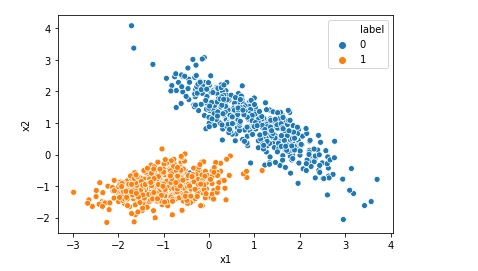# What is Root Mean Squared Error or RMSE

Root mean squared error or RMSE is a measure of the difference between actual values and predicted values of a machine learning model  like Linear Regression. Root mean squared error is a measure of how well the machine learning model can perform. The lower the RMSE, the better the model. RMSE is always positive, and … Continue reading What is Root Mean Squared Error or RMSE# What is Confusion Matrix in Machine Learning

Not only human beings but also the machine learning models may get confused !! After all Artificial Intelligence mimics a human brain, isn’t it?(pun intended). Imagine yourself as a machine learning engineer and suppose you trained a machine learning classification model successfully today. After the model is trained you checked the accuracy which is 93.0%. Wow … Continue reading What is Confusion Matrix in Machine Learning# Difference between Logistic Regression and Support Vector Machine SVM

Both Logistic Regression and Support Vector Machine or SVM can be used for classification however there is some fundamental difference between them. Lets understand those differences when we are using Support Vector Machine as a classifier. Logistic Regression gives probabilities as output that can be interpreted as confidence in the classification decision however Support Vector … Continue reading Difference between Logistic Regression and Support Vector Machine SVM# Top 5 Assumptions of Linear Regression Algorithm

We can not implement Linear Regression on any given data. If we have got a dataset and we are planning to implement Linear Regression Model on that data we must first check if the given data is inline with the assumptions of Linear Regression. Once the given data agrees to all the assumptions then only … Continue reading Top 5 Assumptions of Linear Regression Algorithm

# Top 5 Hyper-Parameters for Logistic Regression

Hyper-parameter is a type of parameter for a machine learning model whose value is set before the model training process starts. Most of the algorithm including Logistic Regression deals with useful hyper parameters. In this post we are going to discuss about the sklearn implementation of hyper-parameters for Logistic Regression. Below is the list of … Continue reading Top 5 Hyper-Parameters for Logistic Regression# Implementing Logistic Regression in 10 lines in Python

Logistic Regression is one of the most popular Machine Learning algorithm used for the classification problems. It should be noted that though there is a regression word in the name of the algorithm Logistic Regression, it is used for classification problems. A use case of Logistic regression could be, based on the symptoms for a disease that a patient has Logistic … Continue reading Implementing Logistic Regression in 10 lines in PythonSupport vector machines or SVM is a supervised machine learning algorithm that can be used for both classification and regression analysis. Advantages of Support Vector algorithm Support vector machine is very effective even with high dimensional data.When you have a data set where number of features is more than the number of rows of data, … Continue reading Top 5 Advantages and Disadvantages of Support Vector Machine Algorithm